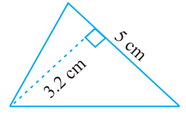# Q. 2.     Find the area of the following triangles:R Riya

We know that

Area of triangle

Here,

Base of triangle = 5 cm

and

Height of triangle =3.2 cm

Therefore, the area of the triangle is

Exams
Articles
Questions